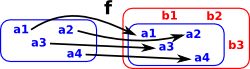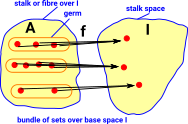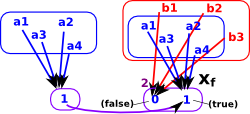# Maths - Sub Object

### Set

Since topos theory is about set-like structures lets look at set first and then generalise to other topoi.

In set all the subobjects are given by the powerset.This set of all subsets comes with some structure (poset structure), the arrows on the above diagram are inclusion maps or we can think of the arrows as meaning 'is a subset of'.

 All the elements of the powerset are subsets of the set {a,b,c}. Also all the elements are supersets of the empty set ø. Therefore all the subsets are representable (in two ways), that is we can replace all the subsets with arrows.### Subobject Classifier

See .

See page here for a non-categorical approach to sub-objects.

 If the arrow 'f' is monic (injective) then it maps to a subobject (subset) of 'B'. That is: there is a subobject of B that is isomorphic to A. The inclusion relation on subobjects is: reflexive transitiveIn order to define the subobject we must define the monic 'upto equality' which is not in the spirit of category theory. We will go on to define a 'subobject classifier' which defines a subobject, in a much more category theoretic way, by the composition of maps (and also relates the whole subject to logic).

 The classifier (characteristic function in subset case)Subset Classifier
ø {0,0,0}
{a} {1,0,0}
{b} {0,1,0}
{c} {0,0,1}
{a,b} {1,1,0}
{a,c} {1,0,1}
{b,c} {0,1,1}
{a,b,c} {1,1,1}

Consider the contravariant functor P : Set->Set which maps each set to its power set and each function to its inverse image map. To represent this functor we need a pair (A,u) where A is a set and u is a subset of A, i.e. an element of P(A), such that for all sets X, the hom-set Hom(X,A) is isomorphic to P(X) via ΦX(f) = (Pf)u = f−1(u). Take A = {0,1} and u = {1}. Given a subset SX the corresponding function from X to A is the characteristic function of S.

#### Naming Arrows

In category theory we don't usually identify elements, such as elements of a set, because we only tend to determine things 'upto isomorphism'. The objects in a category are whole 'structures', they don't represent individual elements.

However, there is the possibility to indicate elements indirectly using arrows. For instance, if we want to enter a specific element we can use:

1->A

because, in sets, 1 is the single element set so it will indicate an element uniquely.

We can also use naming arrows. An arrow:

f: A->B

Is a subset of a function spacef:{0}->BAfis called the 'name' of the function.

#### Classifier

In the opposite direction there is a correspondence between subsets of B and functions:

B->2

This is in the space 2B which corresponds to the powerset(B), that is, all the possible subsets of B.

 The characteristic arrow: xf classifies objects of B to determine if they are images of A. This is a pullback square. The object 2 has two values: 0 and 1. We also use the symbol Ω and call the elements 'true' and 'false'.This means the topos theory is related to logic. (see also 'characteristic function' in number theory).

#### Example in Set

Example in set
Set A (blue) is subset of B (red).If we take the inverse of f we get the concept of a 'bundle' as discussed on this page.classifier for set.## Subobject and Fibre

 Here we discuss combining subobject and fibre (taking part of a fibre).Just to explain the diagram, these two are intended tp be the same: π=(2*I,p1)## Notationthis can be donated as a pair (A,f)

I is common and does not need to be specified.

Where I can, I have put links to Amazon for books that are relevant to the subject, click on the appropriate country flag to get more details of the book or to buy it from them.The Princeton Companion to Mathematics - This is a big book that attempts to give a wide overview of the whole of mathematics, inevitably there are many things missing, but it gives a good insight into the history, concepts, branches, theorems and wider perspective of mathematics. It is well written and, if you are interested in maths, this is the type of book where you can open a page at random and find something interesting to read. To some extent it can be used as a reference book, although it doesn't have tables of formula for trig functions and so on, but where it is most useful is when you want to read about various topics to find out which topics are interesting and relevant to you.